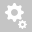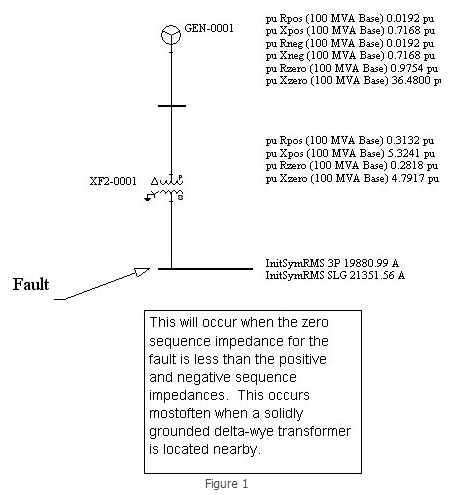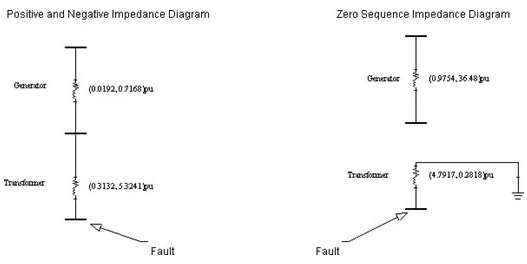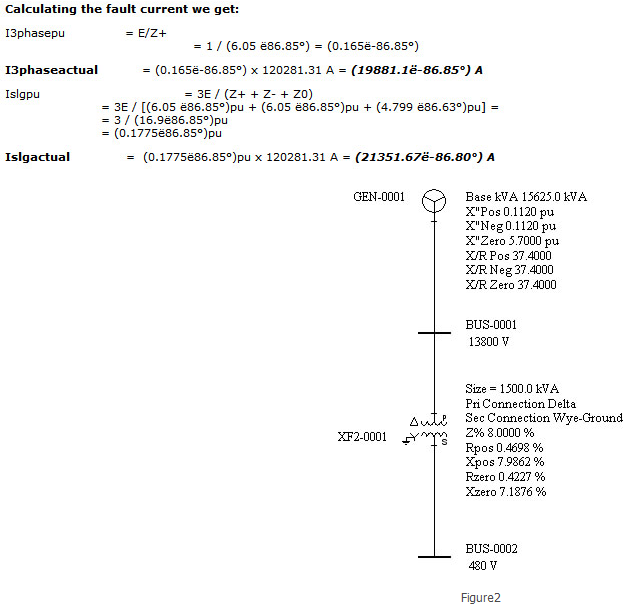##Recently viewed tickets• Sales
• Library Model Request
• Technical Support

# Why is the calculated SLG fault higher than the calculated 3phase fault?

While in most cases it is true that the SLG fault on a bus is lower than the 3Phase fault, there are cases where the SLG fault is higher than 3Phase fault.

This usually occurs when the zero sequence impedance for the fault is less than the positive and negative sequence impedances. This occurs most often when a solidly grounded delta-wye transformer is located nearby.

Figure 1 below is a good example.A sample calculation of the system above follows below. See also Figure 2.

Given:

Generator:

Size: 15625 KVA

X”pos = .1120pu X/R = 37.4 Rpos-> = 0.00299pu

X”neg = .1120pu X/R = 37.4 Rpos-> = 0.00299pu

X”zero = 5.70pu X/R = 37.4 Rpos-> = 0.1524pu

Transformer:

Size: 1500 KVA 13800V/480V D/YG

Rpos = .004698 pu Xpos = 0.079862pu

Rneg = .004698 pu Xneg = 0.079862pu

Rzero = .004227pu Xzero = 0.071876pu

Converting the impedance to 100MVA we get the following:

Zpunew = Zpuold (Sbasenew/Sbaseold)(Vbaseold/Vbasenew)2

Generator:

X”pos = 0.1120pu(100/15.625)(1.0) = 0.7168pu Rpos = 0.00299pu(100/15.625)(1.0) = 0.0192pu

X”neg = 0.1120pu(100/15.625)(1.0) = 0.7168pu Rneg = 0.00299pu(100/15.625)(1.0) = 0.0192pu

X”zero = 5.70pu (100/15.625)(1.0) = 36.48pu Rzero = 0.1524pu (100/15.625)(1.0) = 0.9754pu

Transformer:

Rpos = .004698 pu(100/1.5)(1.0) = 0.3132pu Xpos = 0.079862pu(100/1.5)(1.0) = 5.3241pu

Rpos = .004698 pu(100/1.5)(1.0) = 0.3132pu Xpos = 0.079862pu(100/1.5)(1.0) = 5.3241 pu

Rzero = .004227pu(100/1.5)(1.0) = 0.2818pu Xzero = 0.071876pu(100/1.5)(1.0) = 4.7917 puZ+/-thev = (0.0192, 0.7168)pu + (0.3132, 5.3241)pu= (0.3324, 6.0409)pu = (6.05 ë86.85°)pu

Z0thev = (4.7917, 0.2818)pu = (4.799 ë86.63°)pu

Ibase @ 480V
I = S/VÖ3 = 100MVA / 480 Ö3 = 120281.31 A